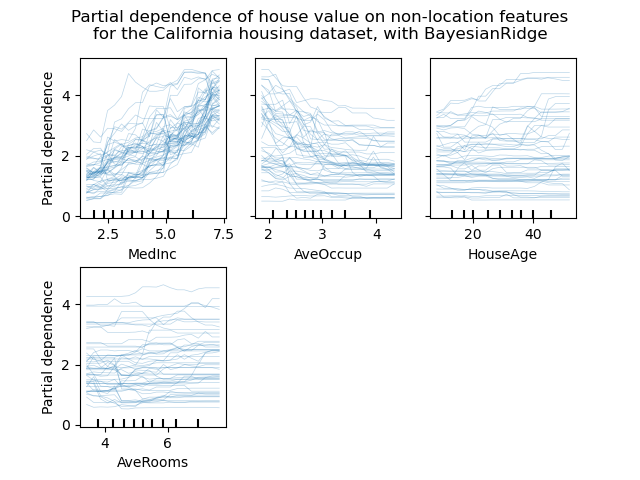# Release Highlights for scikit-learn 0.24¶

We are pleased to announce the release of scikit-learn 0.24! Many bug fixes and improvements were added, as well as some new key features. We detail below a few of the major features of this release. For an exhaustive list of all the changes, please refer to the release notes.

pip install --upgrade scikit-learn


or with conda:

conda install -c conda-forge scikit-learn


## Successive Halving estimators for tuning hyper-parameters¶

Successive Halving, a state of the art method, is now available to explore the space of the parameters and identify their best combination. HalvingGridSearchCV and HalvingRandomSearchCV can be used as drop-in replacement for GridSearchCV and RandomizedSearchCV. Successive Halving is an iterative selection process illustrated in the figure below. The first iteration is run with a small amount of resources, where the resource typically corresponds to the number of training samples, but can also be an arbitrary integer parameter such as n_estimators in a random forest. Only a subset of the parameter candidates are selected for the next iteration, which will be run with an increasing amount of allocated resources. Only a subset of candidates will last until the end of the iteration process, and the best parameter candidate is the one that has the highest score on the last iteration.

Read more in the User Guide (note: the Successive Halving estimators are still experimental).

import numpy as np
from scipy.stats import randint
from sklearn.experimental import enable_halving_search_cv  # noqa
from sklearn.model_selection import HalvingRandomSearchCV
from sklearn.ensemble import RandomForestClassifier
from sklearn.datasets import make_classification

rng = np.random.RandomState(0)

X, y = make_classification(n_samples=700, random_state=rng)

clf = RandomForestClassifier(n_estimators=10, random_state=rng)

param_dist = {
"max_depth": [3, None],
"max_features": randint(1, 11),
"min_samples_split": randint(2, 11),
"bootstrap": [True, False],
"criterion": ["gini", "entropy"],
}

rsh = HalvingRandomSearchCV(
estimator=clf, param_distributions=param_dist, factor=2, random_state=rng
)
rsh.fit(X, y)
rsh.best_params_


Out:

{'bootstrap': True, 'criterion': 'gini', 'max_depth': None, 'max_features': 10, 'min_samples_split': 10}


## Native support for categorical features in HistGradientBoosting estimators¶

HistGradientBoostingClassifier and HistGradientBoostingRegressor now have native support for categorical features: they can consider splits on non-ordered, categorical data. Read more in the User Guide.

The plot shows that the new native support for categorical features leads to fitting times that are comparable to models where the categories are treated as ordered quantities, i.e. simply ordinal-encoded. Native support is also more expressive than both one-hot encoding and ordinal encoding. However, to use the new categorical_features parameter, it is still required to preprocess the data within a pipeline as demonstrated in this example.

## Improved performances of HistGradientBoosting estimators¶

The memory footprint of ensemble.HistGradientBoostingRegressor and ensemble.HistGradientBoostingClassifier has been significantly improved during calls to fit. In addition, histogram initialization is now done in parallel which results in slight speed improvements. See more in the Benchmark page.

## New self-training meta-estimator¶

A new self-training implementation, based on Yarowski’s algorithm can now be used with any classifier that implements predict_proba. The sub-classifier will behave as a semi-supervised classifier, allowing it to learn from unlabeled data. Read more in the User guide.

import numpy as np
from sklearn import datasets
from sklearn.semi_supervised import SelfTrainingClassifier
from sklearn.svm import SVC

rng = np.random.RandomState(42)
random_unlabeled_points = rng.rand(iris.target.shape) < 0.3
iris.target[random_unlabeled_points] = -1
svc = SVC(probability=True, gamma="auto")
self_training_model = SelfTrainingClassifier(svc)
self_training_model.fit(iris.data, iris.target)

SelfTrainingClassifier(base_estimator=SVC(gamma='auto', probability=True))
In a Jupyter environment, please rerun this cell to show the HTML representation or trust the notebook.

## New SequentialFeatureSelector transformer¶

A new iterative transformer to select features is available: SequentialFeatureSelector. Sequential Feature Selection can add features one at a time (forward selection) or remove features from the list of the available features (backward selection), based on a cross-validated score maximization. See the User Guide.

from sklearn.feature_selection import SequentialFeatureSelector
from sklearn.neighbors import KNeighborsClassifier

feature_names = X.columns
knn = KNeighborsClassifier(n_neighbors=3)
sfs = SequentialFeatureSelector(knn, n_features_to_select=2)
sfs.fit(X, y)
print(
"Features selected by forward sequential selection: "
f"{feature_names[sfs.get_support()].tolist()}"
)


Out:

Features selected by forward sequential selection: ['sepal length (cm)', 'petal width (cm)']


## New PolynomialCountSketch kernel approximation function¶

The new PolynomialCountSketch approximates a polynomial expansion of a feature space when used with linear models, but uses much less memory than PolynomialFeatures.

from sklearn.datasets import fetch_covtype
from sklearn.pipeline import make_pipeline
from sklearn.model_selection import train_test_split
from sklearn.preprocessing import MinMaxScaler
from sklearn.kernel_approximation import PolynomialCountSketch
from sklearn.linear_model import LogisticRegression

X, y = fetch_covtype(return_X_y=True)
pipe = make_pipeline(
MinMaxScaler(),
PolynomialCountSketch(degree=2, n_components=300),
LogisticRegression(max_iter=1000),
)
X_train, X_test, y_train, y_test = train_test_split(
X, y, train_size=5000, test_size=10000, random_state=42
)
pipe.fit(X_train, y_train).score(X_test, y_test)


Out:

0.7338


For comparison, here is the score of a linear baseline for the same data:

linear_baseline = make_pipeline(MinMaxScaler(), LogisticRegression(max_iter=1000))
linear_baseline.fit(X_train, y_train).score(X_test, y_test)


Out:

0.7137


## Individual Conditional Expectation plots¶

A new kind of partial dependence plot is available: the Individual Conditional Expectation (ICE) plot. ICE plots visualize the dependence of the prediction on a feature for each sample separately, with one line per sample. See the User Guide

from sklearn.ensemble import RandomForestRegressor
from sklearn.datasets import fetch_california_housing
from sklearn.inspection import plot_partial_dependence

X, y = fetch_california_housing(return_X_y=True, as_frame=True)
features = ["MedInc", "AveOccup", "HouseAge", "AveRooms"]
est = RandomForestRegressor(n_estimators=10)
est.fit(X, y)
display = plot_partial_dependence(
est,
X,
features,
kind="individual",
subsample=50,
n_jobs=3,
grid_resolution=20,
random_state=0,
)
display.figure_.suptitle(
"Partial dependence of house value on non-location features\n"
"for the California housing dataset, with BayesianRidge"
)Out:

/home/circleci/project/sklearn/utils/deprecation.py:87: FutureWarning: Function plot_partial_dependence is deprecated; Function plot_partial_dependence is deprecated in 1.0 and will be removed in 1.2. Use PartialDependenceDisplay.from_estimator instead
warnings.warn(msg, category=FutureWarning)


## New Poisson splitting criterion for DecisionTreeRegressor¶

The integration of Poisson regression estimation continues from version 0.23. DecisionTreeRegressor now supports a new 'poisson' splitting criterion. Setting criterion="poisson" might be a good choice if your target is a count or a frequency.

from sklearn.tree import DecisionTreeRegressor
from sklearn.model_selection import train_test_split
import numpy as np

n_samples, n_features = 1000, 20
rng = np.random.RandomState(0)
X = rng.randn(n_samples, n_features)
# positive integer target correlated with X[:, 5] with many zeros:
y = rng.poisson(lam=np.exp(X[:, 5]) / 2)
X_train, X_test, y_train, y_test = train_test_split(X, y, random_state=rng)
regressor = DecisionTreeRegressor(criterion="poisson", random_state=0)
regressor.fit(X_train, y_train)

DecisionTreeRegressor(criterion='poisson', random_state=0)
In a Jupyter environment, please rerun this cell to show the HTML representation or trust the notebook.